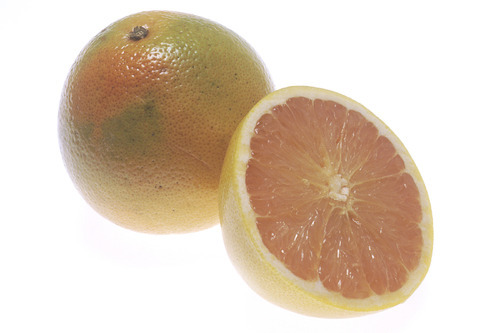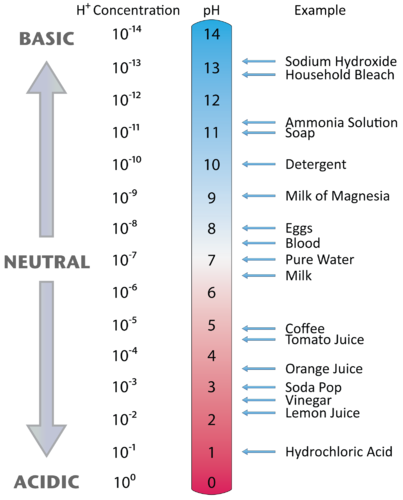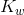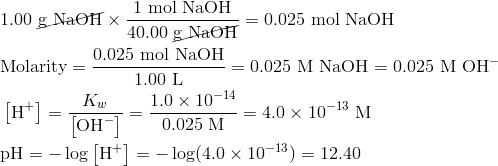The pH Scale

Learning Objectives

• Define pH.
• List pH values of common materials.
• Perform pH calculations for acidic and basic solutions.

Why is grapefruit juice acidic?Grapefruit juice has a pH of somewhere between 2.9-3.3, depending on the specific product. Excessive exposure to this juice can cause erosion of tooth enamel and can lead to tooth damage. The acids in grapefruit juice are carbon-based, with citric acid being one of the major constituents.

This compound has three ionizable hydrogens on each molecule which contribute to the relatively low pH of the juice. Another component of grape juice is malic acid, containing two ionizable hydrogens per molecule.

The pH Scale

Expressing the acidity of a solution by using the molarity of the hydrogen ion is cumbersome because the quantities are generally very small. Danish scientist Søren Sørenson (1868-1939) proposed an easier system for indicating the concentration of H + called the pH scale. The letters pH stand for the power of the hydrogen ion. The pH of a solution is the negative logarithm of the hydrogen-ion concentration.

pH = -log[H + ]

In pure water or a neutral solution the [H + ] = 1.0 × 10 -7  M. Substituting into the pH expression:

pH = -log[1.0 × 10 -7 ] = -(-7.00) = 7.00

The pH of pure water or any neutral solution is thus 7.00. For recording purposes, the numbers to the right of the decimal point in the pH value are the significant figures. Since 1.0 × 10 -7 has two significant figures, the pH can be reported as 7.00.

A logarithmic scale condenses the range of acidity to numbers that are easy to use. Consider a solution with [H + ] = 1.0 × 10 -4  M. That is a hydrogen-ion concentration that is 1000 times higher than the concentration in pure water. The pH of such a solution is 4.00, a difference of just 3 pH units. Notice that when the [H + ] is written in scientific notation and the coefficient is 1, the pH is simply the exponent with the sign changed. The pH of a solution with the [H + ] = 1 × 10 -2  M is 2 and the pH of a solution with the [H + ] = 1 × 10 -10  M is 10.

As we saw earlier, a solution with the [H + ] higher than 1.0 × 10 -7 is acidic, while a solution with the [H + ] lower than 1.0 × 10 -7 is basic. Consequently, solutions whose pH is less than 7 are acidic, while those with a pH higher than 7 are basic. Figure 1 illustrates this relationship, along with some examples of various solutions.Figure 1. The pH values for several common materials.

Calculating pH of Acids and Bases

Raising tropical fishMany people enjoy having tropical fish in their homes or businesses. These brightly-colored creatures are relaxing to watch, but do require a certain amount of maintenance in order for them to survive.

Tap water is usually too alkaline when it comes out of the faucet, so some adjustments need to be made. The pH of the water will change over time while it is in the tank, which means you need to test it every so often. Then you get to be a chemist for your fish.

Calculating pH of Acids and Bases

Calculation of pH is simple when there is a 1 × 10 power problem. However, in real life that is rarely the situation. If the coefficient is not equal to 1, a calculator must be used to find the pH. For example, the pH of a solution with [H + ] = 2.3 × 10 -5  M  can be found as shown below.

pH = -log[2.3 × 10 -5 ] = 4.64

When the pH of a solution is known, the concentration of the hydrogen ion can be calculated. The inverse of the logarithm (or antilog) is the 10 x key on a calculator.

[H + ] = 10 -pH

For example, suppose that you have a solution with a pH of 9.14. To find the [H + ] use the 10 x key.

[H + ] = 10 -pH = 10 -9.14 = 7.24 × 10 -10 M

Hydroxide Ion Concentration and pH

As we saw earlier, the hydroxide ion concentration of any aqueous solution is related to the hydrogen ion concentration through the value of. We can use that relationship to calculate the pH of a solution of a base.

Sample Problem: The pH of a Base

Sodium hydroxide is a strong base. Find the pH of a solution prepared by dissolving 1.0 g of NaOH into enough water to make 1.0 L of solution.

Step 1: List the known values and plan the problem .

Known

• mass NaOH = 1.0 g
• molar mass NaOH = 40.00 g/mol
• volume solution = 1.0 L
• Kw = 1.0 × 10–14

Unknown

• pH of solution = ?

First, convert the mass of NaOH to moles. Second, calculate the molarity of the NaOH solution. Because NaOH is a strong base and is soluble, the [OH ] will be equal to the concentration of the NaOH. Third, useto calculate the [H + ] in the solution. Lastly, calculate the pH.

Step 2: Solve .The solution is basic and so its pH is greater than 7. The reported pH is rounded to two decimal places because the original mass and volume has two significant figures.

Summary

• The concept of pH is defined.
• pH values for several common materials are listed.
• Calculations of pH for acidic and basic solutions are described.

Practice

http://staff.jccc.net/pdecell/chemistry/phscale.html

1. What is a buffer?
2. How much more acidic is vinegar than grapefruit?
3. How much more basic is soapy water than milk of magnesia?

Carry out the requested calculations at the link below:

http://www.sciencegeek.net/APchemistry/APtaters/pHcalculations.htm

Review

1. What is one value of using pH instead of molar concentrations?
2. Is coffee an acidic or a basic substance?
3. If a material has a pH of 9.3, is it acidic or basic?
4. What is the pH of a 4.5 × 10 -3 M HI solution?
5. What is the pH of a 3.67 × 10 -5 M NaBr solution?
6. If we have a weak base with a low ionization constant, can we assume that the [OH ] in the solution is equal to the concentration of the base?

Glossary

• pH: The negative logarithm of the hydrogen-ion concentration.